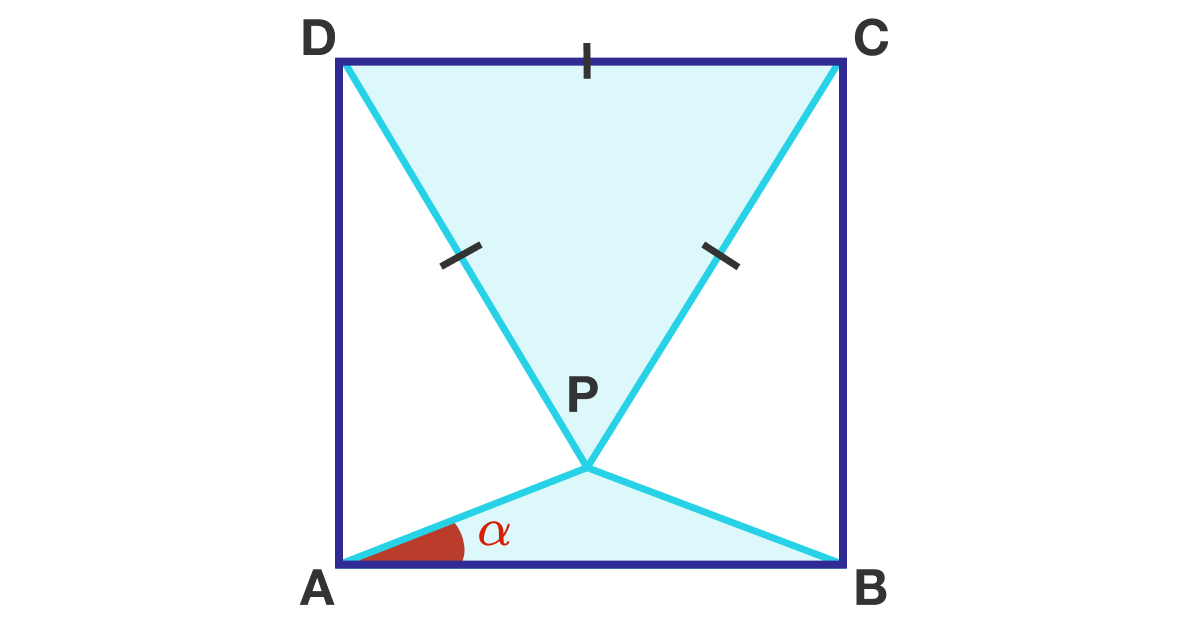# An "Hourglassoid"

Geometry Level 1

Let $ABCD$ be a square, and $P$ a point inside the square such that $\triangle PCD$ is an equilateral triangle, as shown below. Find the angle $\alpha$ in degrees.×